Console.ReadKey() Method makes the program wait for a key press and it prevents the screen until a key is pressed. In short, it obtains the next character or any key pressed by the user. The pressed key is displayed in the console window(if any input process will happen). There are two methods in the overload list of this method as follows:

This method is used to get the next character or function key pressed by the user. The pressed key is displayed in the console window.

Syntax: public static ConsoleKeyInfo ReadKey ();

Return Value: This method returns an object that describes the ConsoleKey constant and Unicode character(if any), it corresponds to the pressed key.

Exception: This method will give InvalidOperationException if the In property is belongs to some stream which is other than the console. “In” property is use to take standard input stream.

Below programs illustrate the use of the above-discussed method:

Example 1:

 `// C# program to illustrate the ` `// Console.ReadKey Method ` `using` `System; ` ` `  `class` `GFG { ` ` `  `    ``// Main Method ` `    ``public` `static` `void` `Main() ` `    ``{ ` `        ``int` `c = 0; ` `        ``Console.WriteLine(``"The series is:"``); ` ` `  `        ``for` `(``int` `i = 1; i < 10; i++)  ` `        ``{ ` `            ``c = c + i; ` `            ``Console.Write(c + ``" "``); ` `        ``} ` ` `  `        ``Console.WriteLine(``"\npress any key to exit the process..."``); ` `     `  `        ``// basic use of "Console.ReadKey()" method ` `        ``Console.ReadKey(); ` `         `  `    ``} ` `} `

Output: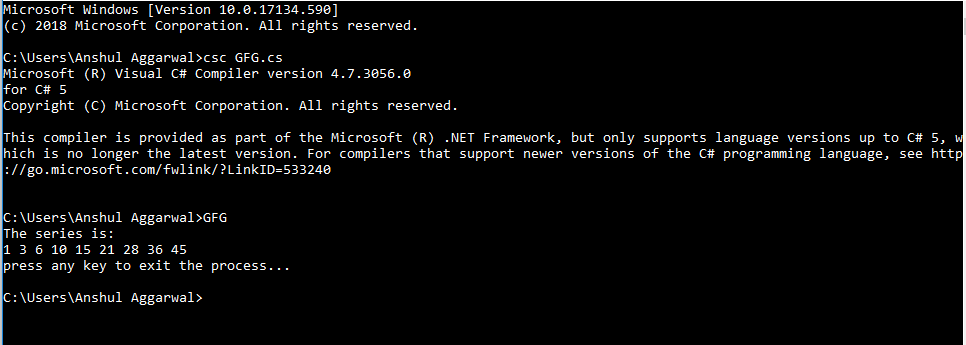Example 2:

 `// C# program to illustrate the ` `// Console.ReadKey Method ` `using` `System; ` ` `  `class` `GFG { ` ` `  `    ``// Main Method ` `    ``public` `static` `void` `Main() ` `    ``{ ` `        ``int` `c = 0; ` `        ``Console.WriteLine(``"The series is:"``); ` `        ``for` `(``int` `i = 1; i < 10; i++)  ` `        ``{ ` `            ``c = c + i; ` `            ``Console.Write(c + ``" "``); ` `        ``} ` ` `  `        ``Console.Write(``"\nPress 'Enter' to exit the process..."``); ` ` `  `        ``// another use of "Console.ReadKey()" method ` `        ``// here it asks to press the enter key to exit ` `        ``while` `(Console.ReadKey().Key != ConsoleKey.Enter) { ` `        ``} ` `         `  `    ``} ` `} `

Output: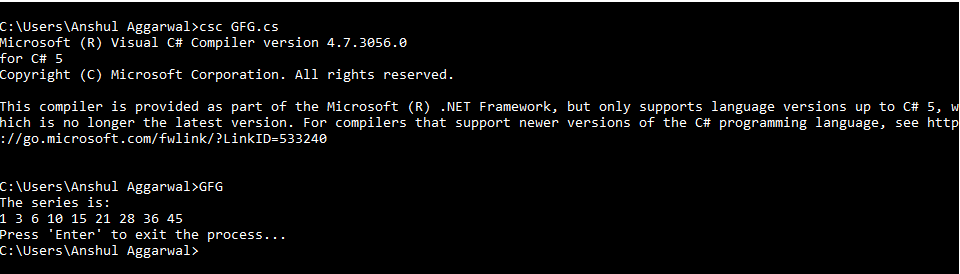Example 3:

 `// C# program to illustrate the ` `// Console.ReadKey Method ` `using` `System; ` ` `  `class` `GFG { ` ` `  `    ``// Main Method ` `    ``public` `static` `void` `Main() ` `    ``{ ` `         `  `        ``// "DateTime" is a inbuilt class  ` `        ``// for date and time ` `        ``DateTime d = DateTime.Now; ` `         `  `        ``// print the system date and time ` `        ``Console.WriteLine(``"System date: {0:d}\n"``+ ` `                        ``"System time: {0:t}"``, d); ` `         `  `        ``Console.Write(``"Press 'E' to exit the process..."``); ` ` `  `        ``// here it ask to press "E" to exit ` `        ``while` `(Console.ReadKey().Key != ConsoleKey.E) { ` `        ``} ` `         `  `    ``} ` `} `

Output: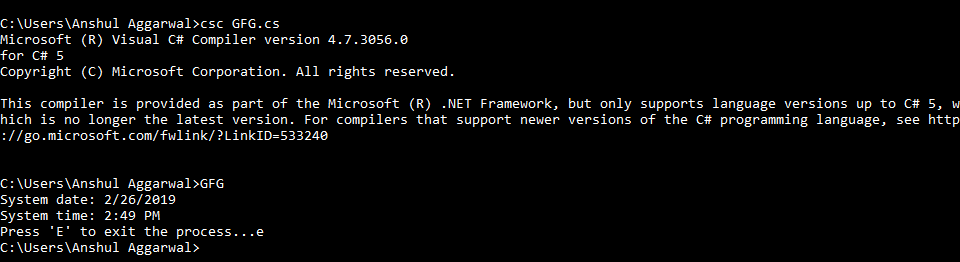This method is more similar to the previous method, that is, it also obtains the next character or any key pressed by the user. The only difference is that the pressed key is optionally displayed in the console window.

Syntax: public static ConsoleKeyInfo ReadKey (bool key);
Here, “key” is used to determines whether to display the pressed key in the console window. If “true” then the pressed key will not be shown in the output window. If “false” then the pressed key will be shown in the output window.

Return Value: This method returns an object that describes the ConsoleKey constant and Unicode character(if any), it correspond to the pressed key.

Exception: This method will give InvalidOperationException when the In property is belongs to some stream which is other than the console. “In” property is use to take standard input stream.

Below programs illustrate the use of the above-discussed method:

Example 1:

 `// C# program to illustrate the  ` `// ReadKey(Boolean) Method ` `using` `System; ` ` `  `class` `GFG { ` ` `  `    ``// Main Method ` `    ``public` `static` `void` `Main() ` `    ``{ ` `        ``int` `c = 0; ` `        ``Console.WriteLine(``"The series is-"``); ` `        ``for` `(``int` `i = 1; i < 10; i++)  ` `        ``{ ` `            ``c = c + i; ` `            ``Console.Write(c + ``" "``); ` `        ``} ` ` `  `        ``Console.WriteLine(``"\npress any key to exit the process..."``); ` ` `  `        ``// here we use "false" in the argument list ` `        ``// when we press any key, the key will  ` `        ``// displays in the console output window ` `        ``Console.ReadKey(``false``); ` `        `  `    ``} ` `} `

Output: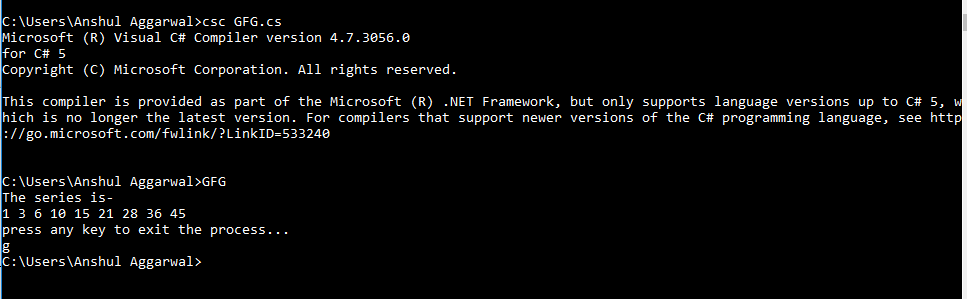Examples 2:

 `// C# program to illustrate the  ` `// ReadKey(Boolean) Method ` `using` `System; ` ` `  `class` `GFG { ` ` `  `    ``// Main Method ` `    ``public` `static` `void` `Main() ` `    ``{ ` `        ``int` `c = 0; ` `        ``Console.WriteLine(``"The series is-"``); ` `        ``for` `(``int` `i = 1; i < 10; i++)  ` `        ``{ ` `            ``c = c + i; ` `            ``Console.Write(c + ``" "``); ` `        ``} ` `        ``Console.Write(``"\nPress 'E' to exit the process..."``); ` ` `  `        ``// here it asks to press "E" to exit ` `        ``// and the key "E" is not shown in ` `        ``// the console output window ` `        ``while` `(Console.ReadKey(``true``).Key != ConsoleKey.E) { ` `        ``} ` `        `  `    ``} ` `} `

Output: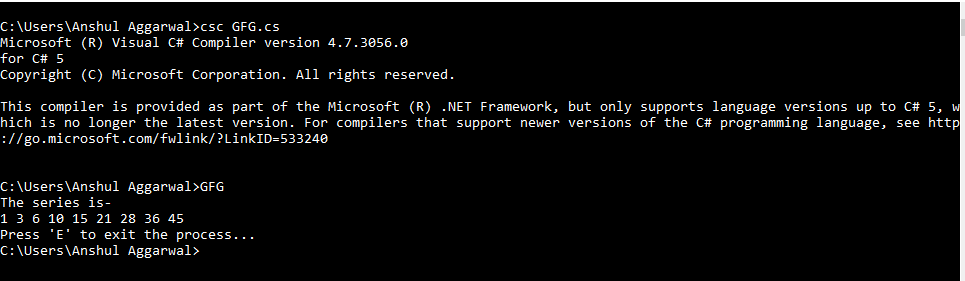Examples 3:

 `// C# program to illustrate the  ` `// ReadKey(Boolean) Method ` `using` `System; ` ` `  `class` `GFG { ` ` `  `    ``public` `static` `void` `Main() ` `    ``{ ` `        ``// "DateTime" is a inbuilt class ` `        ``// for date and time ` `        ``DateTime d = DateTime.Now; ` `         `  `        ``// print the system date and time ` `        ``Console.WriteLine(``"System date: {0:d}\n"``+ ` `                        ``"System time: {0:t}"``, d); ` ` `  `        ``Console.Write(``"Press 'E' to exit the process..."``); ` ` `  `        ``// here it asks to press "E" to exit ` `        ``// The key "E" is shown in the console  ` `        ``// output window because of "false" ` `        ``while` `(Console.ReadKey(``false``).Key != ConsoleKey.E) { ` `        ``} ` `         `  `    ``} ` `} `

Output: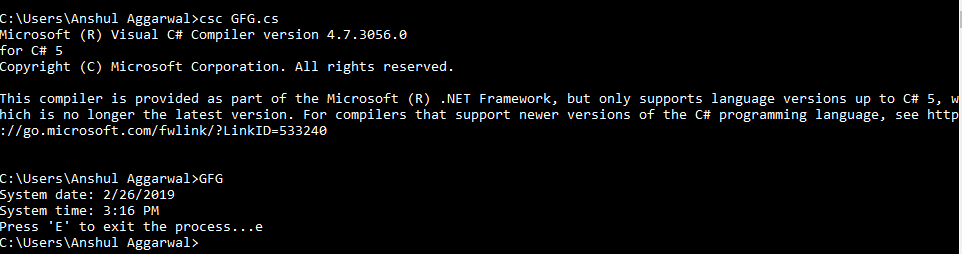My Personal Notes arrow_drop_up3rd year student of Information Technology JADAVPUR UNIVERSITY

If you like GeeksforGeeks and would like to contribute, you can also write an article using contribute.geeksforgeeks.org or mail your article to contribute@geeksforgeeks.org. See your article appearing on the GeeksforGeeks main page and help other Geeks.

Please Improve this article if you find anything incorrect by clicking on the "Improve Article" button below.

Improved By : me53

Article Tags :

1

Please write to us at contribute@geeksforgeeks.org to report any issue with the above content.